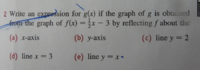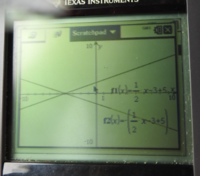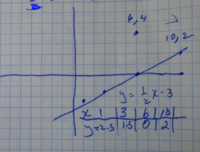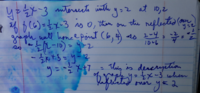# what do they mean here?

#### allegansveritatem

##### Full Member
Here is the problem:I

I am stuck at c). The only thing I could think of doing is adding a 5 to the 1/2x-3 and then putting a minus sign in front of the function for reflecting it. Here is what thhis looks like in calculator window:Is this what they want for c)?
I know that y=2 should be a straight horizontal line but...that doesn't seem to have any purchase here.

#### Jomo

##### Elite Member
If you put red paint on the line (1/2)x-3 and then folded the paper along the line y=2 the result would be that you have a 2nd line on the paper. Do you see that? It is the same line as in your graph. You have one point on this new line. Do you see what it is? Now what is the slope of this line compared to the slope of the line (1/2)x-3 which is 1/2?

Actually you do NOT have the graph of (1/2)x-3. So draw the given line and draw the 2nd line which is reflected across the line y=2

#### tkhunny

##### Moderator
Staff member
Some can be done quite mechanically. I'll show

y = 3x - 4

Reflect across x-axis

-y = 3x - 4

Reflect across y-axis

y = 3(-x) - 4

Reflect across y = x

x = 3y - 4

Think on what those small changes mean to the graph.
Think on what could be done to create the others, reflecting about y = 2 or x = 3.
It may be interesting to find any point that does NOT move at all with the transformation.

Fun example. y = 3. What happens when it is reflected across the y-axis?#### Dr.Peterson

##### Elite Member
I am stuck at c). The only thing I could think of doing is adding a 5 to the 1/2x-3 and then putting a minus sign in front of the function for reflecting it.
In (c), you are asked to reflect the line y = (1/2)x - 3 about the line y = 2.

Have you been taught how to reflect a point, or a function, about a line other than the axis? I suspect so; then you will want to look back at what you were taught, and do it.

I'll assume here that you have to invent it for yourself. One way to do so is to think about what it means to reflect a point about the horizontal line y = b. Given any point (x, y), you want to find a new point (x, y') that is the same distance below y = b as the given point was above y = b. That is, y' - b = -(y - b). Do you see that? Then what does y' have to be, in terms of y and b?

Then try applying that to your equation.

What others have said will help you check that the line you get really is what you want.

#### Harry_the_cat

##### Senior Member
Put aside your GC for a minute.

With paper and pen draw the graph of $$\displaystyle y=\frac{1}{2}x-3$$ ie y-int = -3 and x-int = 6.
Now dot in the line of reflection $$\displaystyle y=2$$.
Now imagine folding the graph along the dotted line, bringing the bottom part up onto the top part.
Draw in the reflected line.

The slope of the original line is 1/2. What is the slope of the reflected line?
The y-int of the original line is -3. What is the y-int of the reflected line?

So now you have the slope and the y-int, what is the equation of the line?

#### allegansveritatem

##### Full Member
If you put red paint on the line (1/2)x-3 and then folded the paper along the line y=2 the result would be that you have a 2nd line on the paper. Do you see that? It is the same line as in your graph. You have one point on this new line. Do you see what it is? Now what is the slope of this line compared to the slope of the line (1/2)x-3 which is 1/2?

Actually you do NOT have the graph of (1/2)x-3. So draw the given line and draw the 2nd line which is reflected across the line y=2
well, one thing I think I can answer: The slope would be the negative of the present line, y=1/2x-3. Thanks for posting. I will get back to this.

Last edited:

#### allegansveritatem

##### Full Member
Some can be done quite mechanically. I'll show

y = 3x - 4

Reflect across x-axis

-y = 3x - 4

Reflect across y-axis

y = 3(-x) - 4

Reflect across y = x

x = 3y - 4

Think on what those small changes mean to the graph.
Think on what could be done to create the others, reflecting about y = 2 or x = 3.
It may be interesting to find any point that does NOT move at all with the transformation.

Fun example. y = 3. What happens when it is reflected across the y-axis?I will have to think about your questions when my noodle comes back to me--sometime tomorrow I hope. But I think I am beginning to see it. Thanks

#### allegansveritatem

##### Full Member
In (c), you are asked to reflect the line y = (1/2)x - 3 about the line y = 2.

Have you been taught how to reflect a point, or a function, about a line other than the axis? I suspect so; then you will want to look back at what you were taught, and do it.

I'll assume here that you have to invent it for yourself. One way to do so is to think about what it means to reflect a point about the horizontal line y = b. Given any point (x, y), you want to find a new point (x, y') that is the same distance below y = b as the given point was above y = b. That is, y' - b = -(y - b). Do you see that? Then what does y' have to be, in terms of y and b?

Then try applying that to your equation.

What others have said will help you check that the line you get really is what you want.
I really don't think I recall having been introduced to this type of procedure before. I know how to reflect across the axes but not otherwise...but I am getting a glimmer of what would be entailed. Somehow the two lines will intersect on the line to be reflected over...So, if I can find the point of intersection I can draw the second graph through it...Am I right? I will have to study your post tomorrow. No head for it now. Very late. Thanks

#### allegansveritatem

##### Full Member
Put aside your GC for a minute.

With paper and pen draw the graph of $$\displaystyle y=\frac{1}{2}x-3$$ ie y-int = -3 and x-int = 6.
Now dot in the line of reflection $$\displaystyle y=2$$.
Now imagine folding the graph along the dotted line, bringing the bottom part up onto the top part.
Draw in the reflected line.

The slope of the original line is 1/2. What is the slope of the reflected line?
The y-int of the original line is -3. What is the y-int of the reflected line?

So now you have the slope and the y-int, what is the equation of the line?
I don't think I know what y-int means...maybe I do but if so what I know is not thus designated. I will look it u p. Thanks for posting

#### Dr.Peterson

##### Elite Member
I really don't think I recall having been introduced to this type of procedure before. I know how to reflect across the axes but not otherwise...but I am getting a glimmer of what would be entailed. Somehow the two lines will intersect on the line to be reflected over...So, if I can find the point of intersection I can draw the second graph through it...Am I right? I will have to study your post tomorrow. No head for it now. Very late. Thanks
That would be one way to do it in this case; it wouldn't be sufficient if, say, the graph you were reflecting didn't intersect the line you are reflecting over. But there are other ways besides my suggestion. In the end, there is a fairly simple formula you can use.

#### allegansveritatem

##### Full Member
I worked on this again today. I am posting here the program that I followed (I used it for for d) and e) as well).
In short, what I did was graph the line given, find the point at which it intersects with y=2, find another point that is on the other side of y=2 that corresponds with 6,0 but shifted up 4 and then use those two points to find the slope of the reflected graph (I knew this already but...) and then use the point-slope form to find the equation of reflected line. Is this the most streamlined strategy? Probably not, but it is what I hit upon.#### Harry_the_cat

##### Senior Member
Yes that's correct. Well done.

BTW, y-int means y-intercept, ie where the graph cuts the y-axis.

If the equation of a line is given in y = mx + c form, then c is the y-int and is the gradient.

What you've done is correct and is a valid method, but if you want a more "stream-lined" way, reread my post #5.

#### Dr.Peterson

##### Elite Member
Now I can tell you my method as suggested in post #4.

Given a point (x,y) and line y=b, the reflected point (x,y') will satisfy y' - b = -(y - b), as far above b as y is below. Simplifying this, you get y' = 2b - y. This amounts to reflecting across the x-axis (-y) and then shifting up 2b (+ 2b).

So you want to do that to your line y = (1/2)x - 3: reflect it across the axis to get y = -(1/2)x + 3, and then shift up by 4 (twice 2) to get y = -(1/2)x + 7.

Or, just plug it into my formula: y = 2*2 - [(1/2)x - 3] = 4 - (1/2)x + 3 = -(1/2)x + 7.

I don't know what method you are expected to use, not having been taught anything about it.

#### Harry_the_cat

##### Senior Member
I agree that there are various methods to solve this sort of problem. I don't think you need to be taught specifically how do this.
If you have knowledge of:
(1) straight lines and their equations
(3) intercepts and/or intersection points
(4) concept of reflection,
then you should aim to be able to work it out for yourself.

#### allegansveritatem

##### Full Member
Yes that's correct. Well done.

BTW, y-int means y-intercept, ie where the graph cuts the y-axis.

If the equation of a line is given in y = mx + c form, then c is the y-int and is the gradient.

What you've done is correct and is a valid method, but if you want a more "stream-lined" way, reread my post #5.
well, when you used the abbreviation "int" I didn't associate it with the word intercept, probably because my calculator uses "int" to stand for, among other things, greatest integer function. I did reread the post and I think it influenced to some extent how I proceeded here.

•Harry_the_cat

#### allegansveritatem

##### Full Member
Now I can tell you my method as suggested in post #4.

Given a point (x,y) and line y=b, the reflected point (x,y') will satisfy y' - b = -(y - b), as far above b as y is below. Simplifying this, you get y' = 2b - y. This amounts to reflecting across the x-axis (-y) and then shifting up 2b (+ 2b).

So you want to do that to your line y = (1/2)x - 3: reflect it across the axis to get y = -(1/2)x + 3, and then shift up by 4 (twice 2) to get y = -(1/2)x + 7.

Or, just plug it into my formula: y = 2*2 - [(1/2)x - 3] = 4 - (1/2)x + 3 = -(1/2)x + 7.

I don't know what method you are expected to use, not having been taught anything about it.
That is a good way and I think I was on the point of sniffing it out today but didn't quite get clear in my mind how to set it into a formula--I knew there was a formula floating around somewhere but couldn't grab it.

#### allegansveritatem

##### Full Member
I agree that there are various methods to solve this sort of problem. I don't think you need to be taught specifically how do this.
If you have knowledge of:
(1) straight lines and their equations
•